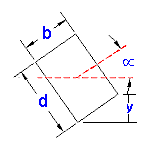Area Moment of Inertia Section Properties: Rectangle at Specified Angle

Area Moment of Inertia Section Properties: Rectangle at Specified Angles

Area Moment of Inertia Section Properties of Rectangle at Specified Angles Calculator and Equations. This engineering calculator will determine the section modulus for the given cross-section. This engineering data is often used in the design of structural beams or structural flexural members.

 Description Equation Area Moment of Inertia Section Properties = ISection Modulus = Z = I/yRadius of GyrationA = area A = db y = Distance to neutral axisArea Moment of Inertia Section Properties: Rectangle at Specified Angle
 Variables Inputs Inches mm "b" Width = "d" Height = "??" Angle = Calculated Properties Inches mm Area Moment of Inertia Section Properties (Unit4) = Section Modulus (Unit3) = Radius of Gyration (Unit) = Area (Unit2) = y = (unit) =Membership Register | LoginHomeEngineering Book StoreEngineering ForumExcel App. DownloadsOnline Books & ManualsEngineering NewsEngineering VideosEngineering CalculatorsEngineering ToolboxGD&T Training Geometric Dimensioning TolerancingDFM DFA TrainingTraining Online EngineeringAdvertising CenterCopyright Notice